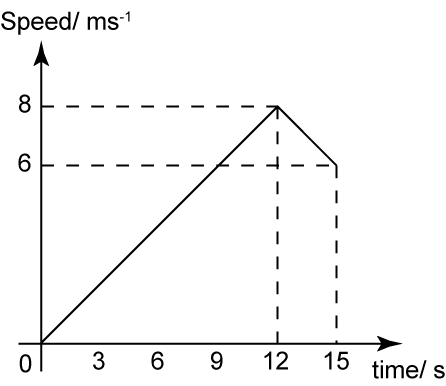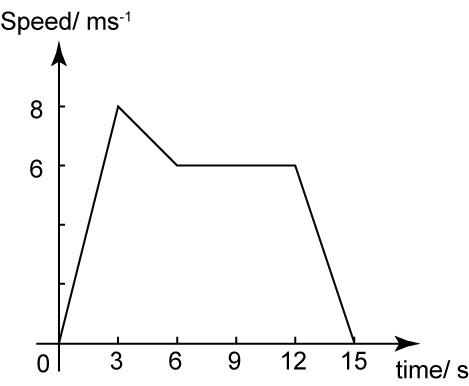# 6.3.3 Gradient and Area Under a Graph, SPM Paper 2 (Long Questions)

Question 7:The diagram above shows the speed-time graph of a moving object for 15 seconds. Find
(a)    the speed of the object at t = 9s.
(b)   the distance travel by the object for the first 12 seconds.

Solution:

(a)
The speed of the object at t = 9s is 6 ms-1

(b)
Distance travel by the object for the first 12 seconds
= Area under the speed-time graph
= Area of triangle
= ½ × 12 × 8
= 48 m

Question 8:The diagram above shows the speed-time graph of a moving object for 15 seconds.
(a) Find the time interval when the object moves with constant speed.
(b) Find the acceleration from t = 6 s to t = 12 s.
(c) State the time when the object is stationary.

Solution:

(a)
Time interval when the object moves with constant speed
= 12 – 6
= 6 s

(b)

(c)

Time when the object is stationary is at 15 s.

Question 9:
Diagram below shows the speed-time graph for the movement of an object for a period of 34 seconds.(a) State the duration of time, in seconds, for which the object moves with uniform speed.
(b) Calculate the rate of change of speed, in ms-2, of the object for the first 8 seconds.
(c) Calculate the value of u, if the average of speed of the object for the last 26 seconds is 6 ms-1.

Solution:
(a) Duration of time = 26s – 20s = 6s